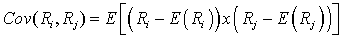Tags
Question
Given two random variables, Ri and Rj, the covariance between the two variables is:

Cov (Ri,Rj)= [...]Tags
Question
Given two random variables, Ri and Rj, the covariance between the two variables is:

Cov (Ri,Rj)= [...]
?

Tags
Question
Given two random variables, Ri and Rj, the covariance between the two variables is:

Cov (Ri,Rj)= [...]If you want to change selection, open document below and click on "Move attachment"

Subject 7. Covariance and Correlation
, what is the relationship between the performance of the S&P 500 and that of U.S. long-term corporate bonds? We can use covariance and correlation to measure the degree to which two random variables are related to each other. <span>Given two random variables, R i and R j , the covariance between the two variables is: Facts about covariance: Covariance of returns is negative if, when the return on one asset is above its expected value, the return o

#### Summary

status measured difficulty not learned 37% [default] 0

No repetitions